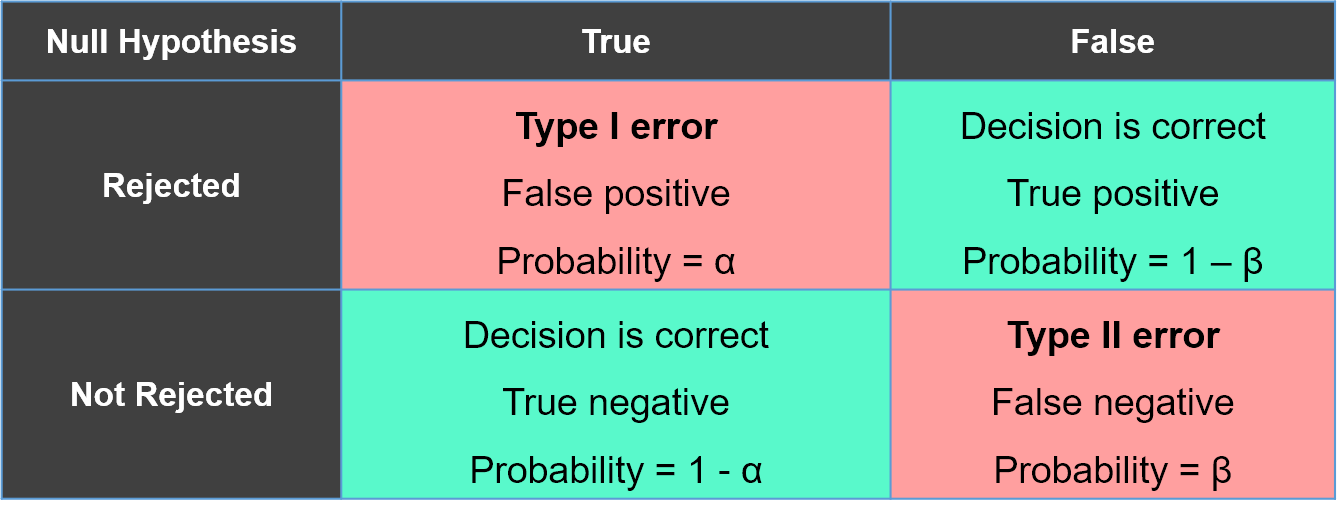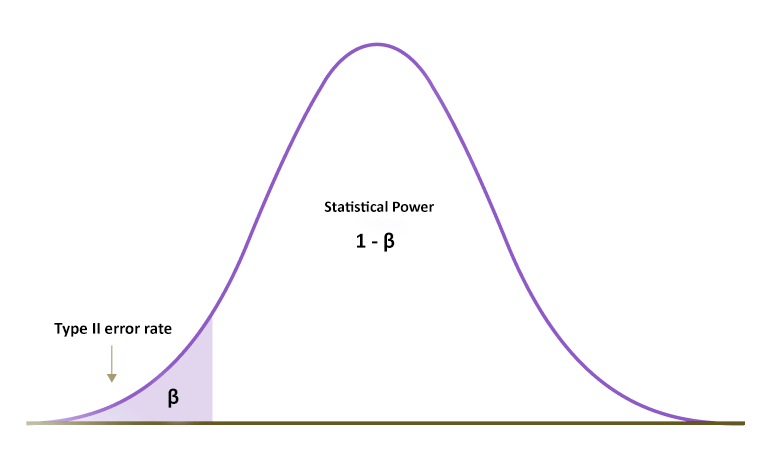# What are Type I and Type II errors? How to avoid them?

Easy Last updated on May 7, 2022, 1:32 a.m.

Errors in statistics are important metrics for making any statistical decision. A statistical decision almost always involves uncertainties, and understanding the risks and the effect of the errors is vital to conclude any statistical testing.

Before diving in, we suggest you go over P-value (Hypothesis testing) article. To understand Type I and Type II errors, lets’ go over an example:

Let us say that a person A wants to get tested for COVID-19. This person might have contracted the virus and showed mild symptoms, or it could just be a rapid change in the weather!

Here is what the errors would look like:

Type I error: Test says are COVID positive, but person A actually is not!

Type II error: Test says you don’t have COVID but person A actually does!

Here, the Type I error represents a false positive situation, whereas the Type II error indicates a false negative situation!Fig 1: Illustration of Null Hypothesis vs Errors. Alpha($\alpha$) is the probability of making a Type I error and beta (β) is the probability of making a Type II error.

Let us dive into each of these errors to understand them in greater detail.

### Type I error (we reject the null hypothesis when it is actually true):

Type I error, also known as a “false positive,” occurs when we observe a difference when in truth, there is none (in technical terms - no statistically significant difference). The probability($\alpha$) of making a type I error in a test with rejection region R(refer fig 2) is P($R |H_0$ is true).

when we say that the significance level is 5% or 0.05, the results only have a 5% chance of occurring, considering that the null hypothesis is true.

For Example, In a study, if the p-value obtained is 0.045 and the level of significance is 5%, it is clear that the given p-value is less than the level of significance, which is 0.05. This means that we reject the null hypothesis but looking at the p-value, we can still conclude that there is a 4.5% chance of the result occurring if the null hypothesis was true. This indicates that there is a risk of making a Type I error!

### Type II error (we fail to reject the null hypothesis, due to insignificant evidence):

Type II error, also known as a “false negative,” occurs when the study conducted may not have enough statistical relevance or power to actually understand the effect. In other words, we fail to observe a difference when in truth, there is one. So the probability($\beta$) of making a type II error in a test with rejection region R is $1- P(R|H_0$ is true) , and the statistical power($1-\beta$) of test can be written as $P(R|H_0$ is true) .

Statistical power is the extent to which an experiment conducted can detect a real effect correctly when in truth, there is one. A power level equal to or higher than 80% is considered statistically acceptable in many scenarios. However, the risk of a Type II error is inversely proportional to the statistical power of an experiment. So, the lower the statistical power, the higher the probability of a Type II error!

Here’s how we can determine statistical power:

• Significance level($\alpha$): Increasing significance level increases statistical power.
• Effect size: Effect Size is defined as the mean difference relative to the standard deviation of the variable within the population.
$$Effect Size(d) = (\mu_{true} -\mu_{hypo})/ \sigma$$
Larger effects can be more easily detected.
• Sample size: Increasing the sample size decreases the likely difference between the true population mean and the mean of your sample. Larger sample sets increase power by reducing sampling errors.

To reduce the risk of a Type II error, we can increase the significance level or increase the sample size in consideration!Fig3: Here, the Type II error rate is beta (β) is represented in the shaded area on the left side. All the remaining area in the curve represents the statistical power, 1 - β.

## How to avoid them?

We can decrease the possibility of Type I error by reducing the significance level. Still, to minimize a Type II error occurrence, we need to increase the significance level for that experimentation!

In a real-world setting, Type I errors are more complicated to handle than Type II errors because Type I error means that we are going against the primary statistical assumption of the actual null hypothesis, which in turn may create new policies or practices that can be considered a waste of resources. On the other hand, Type II error means we fail to reject the null hypothesis. At worst, this may lead to missed opportunities for innovation, but the consequences are far less severe than Type I error.

References